## Proof of Conservation of Angular Momentum

Theorem

Angular momentum is conserved for a body subject to no net external torque.

Proof

Let a body consist of particles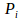at position vectors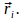The motion of each particle is determined by Newton's second law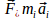or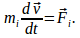Taking the cross product with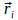and summing over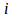gives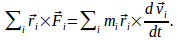Now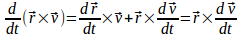since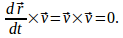We can write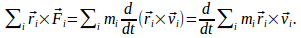The right hand side of this equation is the rate of change of angular momentum. Consider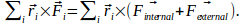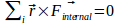by Newton's third law so if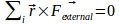then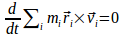and angular momentum is conserved.